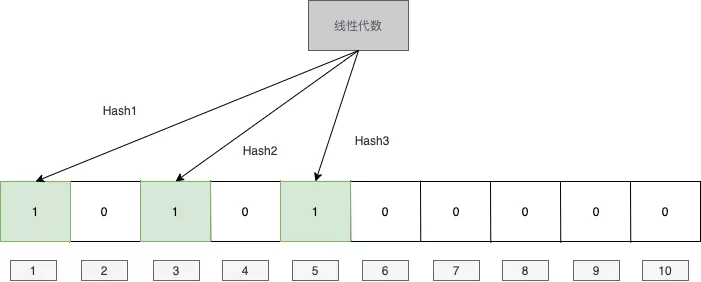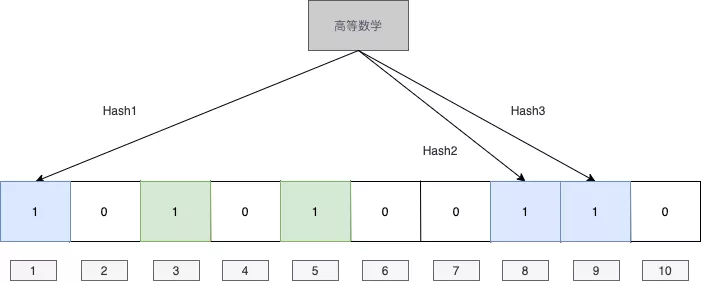# 大數據算法——布隆過濾器

## 原理## 代碼

# 插入元素
def BloomFilter(filter, value, hash_functions):
m = len(filter)
for func in hash_functions:
idx = func(value) % m
filter[idx] = True
return filter

# 判斷元素
def MemberInFilter(filter, value, hash_functions):
m = len(filter)
for func in hash_functions:
idx = func(value) % m
if not filter[idx]:
return False
return True


## 錯誤率計算

$\displaystyle\left[1-(1-\frac{1}{m})^{nk}\right]^k \approx (1-e^{-\frac{kn}{m}})^k$

$\displaystyle\lim_{x \to -\infty}(1-\frac{1}{x})^{-x}=e$

$f(k) = (1-b^{-k})^k \\ \ln f(k) = k\ln(1-b^{-k})$

\begin{aligned} \frac{1}{f(k)}f'(k)&= ln(1-b^{-k}) + \frac{kb^{-k}\ln b}{1-b^{-k}} \end{aligned}

\begin{aligned} \ln(1-b^{-k})(1-b^{-k})&=-kb^{-k}\ln b\\ \ln(1-b^{-k})(1-b^{-k})&=b^{-k}\ln b^{-k}\\ 1-b^{-k} &=b^{-k}\\ b^{-k} &= \frac{1}{2} \end{aligned}

## 靈魂拷問posted @ 2020-02-15 09:22  TechFlow2019  閱讀(7237)  評論(21編輯  收藏